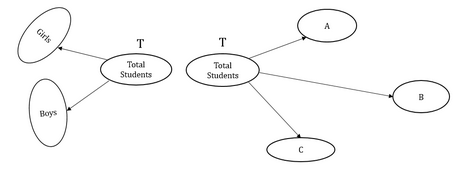Courses

# If the students were awarded only three grades-A, B and C-how many students in the class got grade A?(1) 20% of the class got grade B and 50% of the class got grade C. (2) The 12 girls in the class make up 40% of the class. a+b+c=Ta)Statement (1) ALONE is sufficient, but statement (2) alone is not sufficient.b)Statement (2) ALONE is sufficient, but statement (1) alone is not sufficient. c)BOTH statements TOGETHER are sufficient, but NEITHER statement ALONE is sufficient. d)EACH statement ALONE is sufficient. e)Statements (1) and (2) TOGETHER are NOT sufficient.Correct answer is option 'C'. Can you explain this answer? Related Test: Test: Tree Structure

## GMAT Question

By Rajat Katiyar · Apr 23, 2020 ·GMAT
Future Foundation Institute answered 4 weeks agoSteps 1&2: Understand question and draw inferences
This question involves the creation of two trees.
In the first tree, the node ‘Total Students’ is branched on the basis of gender. So, we get two branches: Girls and Boys.
Let the number of Girls in the class be g, and the number of boys in the class be b.
Let the total number of students in the class be T.
So, we can write:
g+b=T
. . . Equation 1
In the second tree, the node ‘Total Students’ is branched out on the basis of grades.
Let the number of students who got grades A, B and C be a, b and c respectively.
So, we can write:
a+b+c=T. . . Equation 2
We need to find the value of a.
Step 3: Analyze Statement 1
As per Statement 1,
20% of the class got grade B and 50% of the class got grade C
b = 20% of T  and c = 50% of T
⇒ b = 0.2T and c = 0.5T
Substituting these values of b and c in Equation 2:
a + 0.2T + 0.5T = T
a=0.3 T
Since we don’t know the value of T, we will not be able to find the value of a.
Therefore, Statement 1 is not sufficient.
Step 4: Analyze Statement 2
As per Statement 2,
The 12 girls in the class make up 40% of the class
⇒ g=12
and g=40% of T
⇒  12 = 40100T
⇒ T = 30
However, this statement gives us no clue about the value of a. Therefore, this statement is clearly not sufficient.

Step 5: Analyze Statement 1 and Statement 2 together (if needed)
From Statement 1,
a=0.3 T
From Statement 2,
T = 30
Thus, by combining the two statements,
We get a = 9
Thus, we are able to get a unique value by combining the two statements.

Simple analysis to be done ,don’t judge it be very high level.
ITS A Qn of how many got A,THAT MEANS EXACT VALUE TO BE FOUND TO GET THIS KIND OF DS QNS.

STATEMENT-1 INDIRECTLY SAYS 30% OF CLASS GOT A GRADE,BUT DO U KNOW FROM THE 1ST STATEMENT THE CLASS STRENGTH? NO.  SO NOT SUFFICIENT
STATEMENT-2 SAYS 12 GIRLS MAKE 40% CLASS STRENTH,SO 100% OF CLASS CAN BE CALCULATED,BUT WITH THIS STATEMENT ALONE I CANT KNOW HOWMANY PEOPLE GET A GRADE,SO NOT SUFFICIENT.

COMBINE FROM ST-2 WE CAN KNOW 100% class strength , and using ST-1 ,I CAN CALCULATE 30% OF THAT CLASS .

DONT CALCULATE ,JUST IMAGINATION IS REQUIRED.